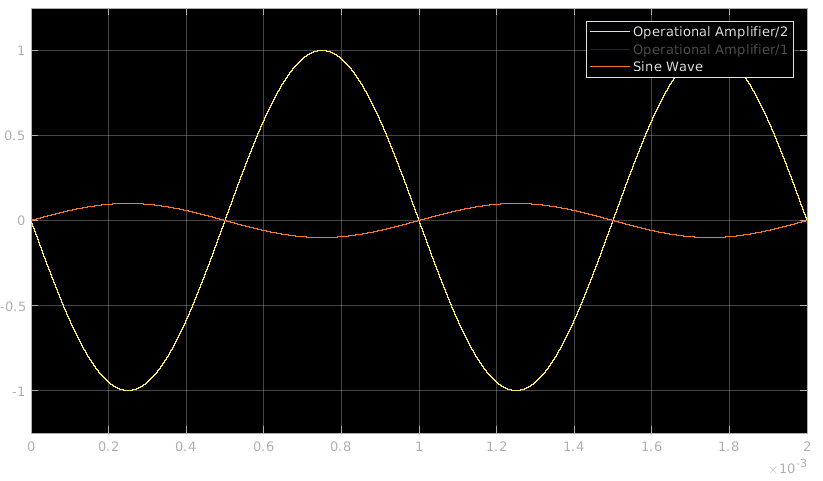This example shows how to model an inverting amplifier circuit using the Operational Amplifier block and the Linear Circuit wizard block.

### Inverting Amplifier

The inverting amplifier circuit contains an op-amp and a feedback network consisting of passive resistors. In this example, the Linear Circuit Wizard block is used to model the feedback network. The Linear Circuit Wizard uses a SPICE netlist describing the feedback stage from the output of the op-amp to its input.

Open the model `Inverting_Amplifier` attached to this example.

```open_system('Inverting_Amplifier'); ```### Operational Amplifier

The op-amp used in this example is a double pole circuit defined from the circuit parametes. In this example, the Input offset voltage (V) is set to `0`, the Output resistance (Ohms) is set to `80`, and the Open loop gain (v/v) is set to `855e3`. The Unity Gain Bandwidth (Hz) is `1e8` Hz and the Maximum Tail Current (A) is `100e-6`.

### Linear Circuit Wizard

The Linear Circuit Wizard block uses a SPICE netlist to generate a linear circuit block. You can specify the SPICE netlist file name and click on the Build/modify block button to create a circuit block that models the SPICE netlist. The netlist attached to this example is InvertingAmplifier.sp.

### Operation

The inverting amplifier circuit is designed with an Op-amp and a feedback network consisting of two resistors R2 and R1.The feedback from Op-amp output to the input terminal is modeled in the attached SPICE file (R2 = 20KOhms, R1 = 2KOhms).

The closed loop gain of the inverting amplifier is -R2/R1, which is equal to -10. The input stimulus applied to this model has a sample rate of `1e-8`. The same sample rate is used in the operational amplifier.

An input signal of 0.1V sine wave with 1KHz frequency is provided to the circuit model. The input and corresponding output are seen on the scope.